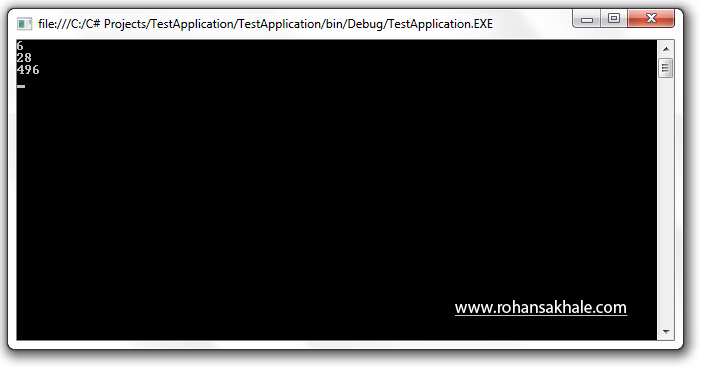# Program to find Perfect Number's upto 1000

3/12/2012 code-examplecsharp

### # Summary

Perfect Number is a positive number that is equal to the sum of its proper divisors.

### # Example

6 is a perfect number since its divisors are 1,2,3 and 1 + 2 + 3 = 6.

### # Screenshot### # Code

``````using System;

namespace TestApplication
{
class PerfectNumber
{
public static void Main()
{
int numbers = 1000;
int sum;
for(int i = 1;i <= numbers; i++)
{
sum = 0;
for (int j = 1; j < i; j++)
{
if (i % j == 0)
{
sum = sum + j;
}
}
if (i == sum)
{
Console.WriteLine(i);
}
}
}
}
}
``````
1
2
3
4
5
6
7
8
9
10
11
12
13
14
15
16
17
18
19
20
21
22
23
24
25
26
27
28
29